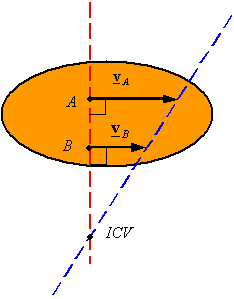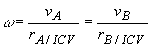Instantaneous Center of Velocity

Extension of a rigid body: The extension of a rigid body refers to the operation of theoretically extending the body to fill all space. By this operation every point in space becomes a point of the body and as a result has a velocity associated with it. Since this is not an actual extension of the body, a theoretical extension does not influence how the actual body moves-it simply follows the motions of the actual body.

Instantaneous Center of Velocity (ICV): Any point on a rigid body or on its extension that has zero velocity is called the Instantaneous Center of Velocity of the body. Assuming one knows the ICV of a body, one can calculate the velocity of any point A on the body using the equation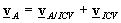and recognizing that be definition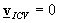. This gibes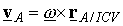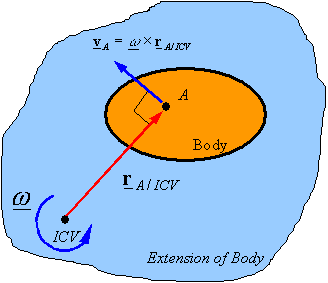In 2-D motion, if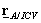is in the plane of motion and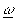is perpendicular to this plane, then one can use the scalar relation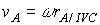Methods of finding the ICV: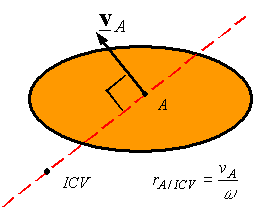·        Given the velocity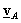of point A on a rigid body and the angular velocity of the rigid body one can use the above equation to find the distance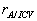between the point A and the ICV. One can then draw a line perpendicular to the velocity and passing through A, and move along this line a distanceto get to the ICV. The side on which the ICV is can be determined by the direction of the angular velocity.

·        Given the velocity of points A and B on a rigid body one can find the ICV by drawing a line perpendicular toand passing through A, and by drawing a line perpendicular to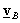and passing through B. One of the following three cases will result

·        The lines intersect at one point: The point of intersection is the ICV. The angular velocity can be calculated once the ICV is determined using the velocity of either point and its corresponding distance from the ICV.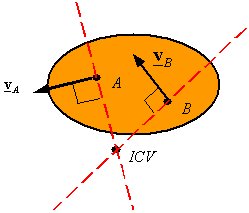·        The lines are parallel (they intersect at infinity): The ICV is at infinity, and the angular velocity is zero since infinity times zero is the only way one can get velocities other than infinite. Therefore, the body is in pure translation and the velocity of the two points must be the same.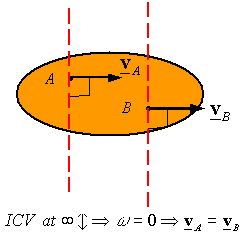·        The two lines fall on top of each other: One can find the location of the ICV using the proportionality of velocity and distance from the ICV to create similar triangles. This follows from